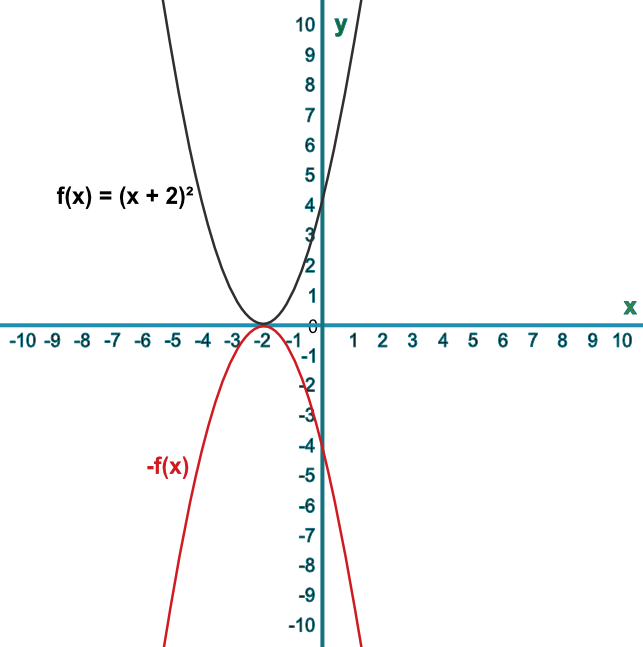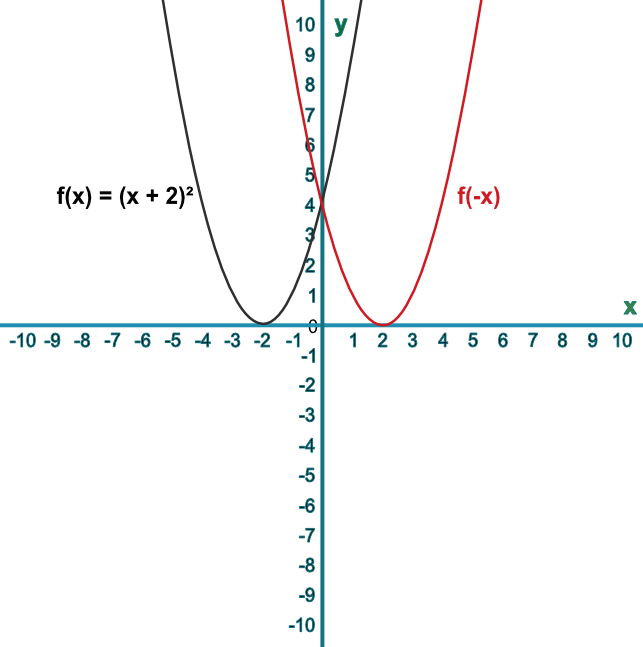Sketch Reflections of Functions

## Sketch Reflections of Functions

Graphs can be reflected by applying negative values to a function.

If the negative value is applied before the function takes place, then the result is a reflection in the y-axis.

If the negative value is applied to the result of the function, then the reflection will take place in the x-axis:

## Example 1

Sketch the graph of the function f(x)=x^2. On the same graph, sketch -f(x).

Describe the transformation.

A negative value applied after the function is evaluated will reflect the curve in the x-axis.

Answer: The curve is reflected in the x-axis.## Example 2

Sketch the graph of the function f(x)=x^2. On the same graph, sketch f(-x).

Describe the transformation.

A negative value applied before the function is evaluated will reflect the curve in the y-axis.

Answer: The curve is reflected in the y-axis.